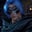Related Tags

perl
array
size
communitycreator

# How to find the size of an array in PerlGutha Vamsi Krishna

An array variable starts with an @ in PERL, while a scalar variable begins with a $. We have to manipulate the array to get its size. This can be done using either of the following two ways: 1. Implicit scalar conversion 2. Explicit scalar conversion Let's take a look at these. ### What is implicit scalar conversion? We can get the size of an array by simply assigning a scalar variable to the array, and the variable will hold the size of the given array. This process is called implicit scalar conversion. Let's see how this looks like in the code example below. # given array @nums = (10,22,39,44); # get size of the array$length = @nums;

#display the size of the array
print \$length;
Example for Implicit scalar conversion

### Explanation

In the above code snippet, we do the following:

• Line 2: We declare and initialize an array nums.
• Line 5: We get the size of the given array nums by assigning the scalar variable length to the array nums.
• Line 8: We display the variable length, which holds the size of the array nums.

### What is explicit scalar conversion?

We can get the size of an array by using the scalar context of the array. We can simply achieve this by adding the scalar keyword while printing the array. Explicitly telling our program to print the scalar value is called explicit scalar conversion.

We can see it working in the code below:

# given array
@nums = (10,22,39,44);

#display the size of the array
print scalar @nums;
Explicit scalar conversion

### Explanation

In the above code snippet, we do the following:

• Line 2: We declare and initialize an array nums.
• Line 5: We display the size of the array nums, which is returned by scalar @nums.

RELATED TAGS

perl
array
size
communitycreator

CONTRIBUTORGutha Vamsi Krishna
RELATED COURSES

View all Courses

Keep Exploring

Learn in-demand tech skills in half the time